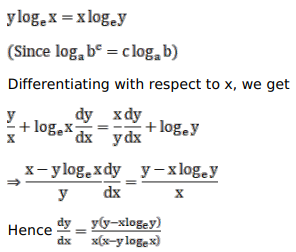# Solve this following

Question:

Mark $(\sqrt{ })$ against the correct answer in the following:

If $x^{y}=y^{x}$ then $\frac{d y}{d x}=$ ?

A. $\frac{(y-x \log y)}{(x-y \log x)}$

B. $\frac{y(y-x \log y)}{x(x-y \log x)}$

C. $\frac{y(y+x \log y)}{x(x+y \log x)}$

D. none of these

Solution: